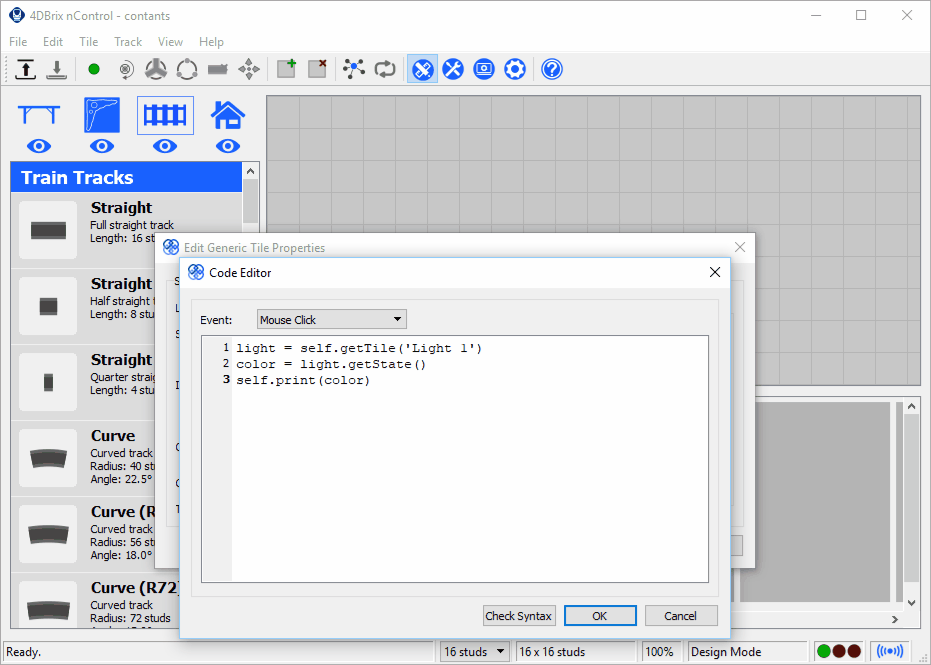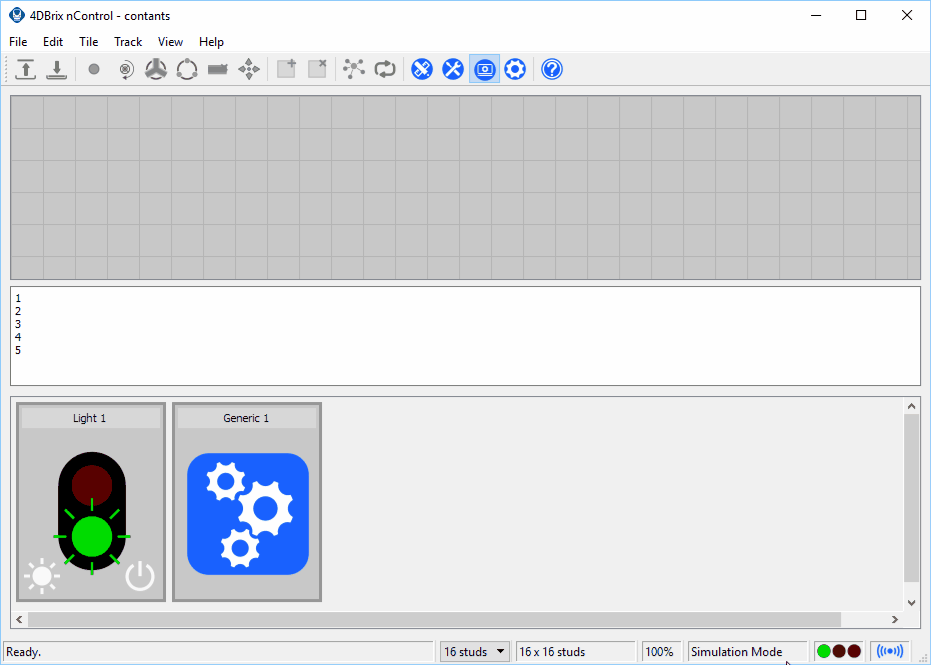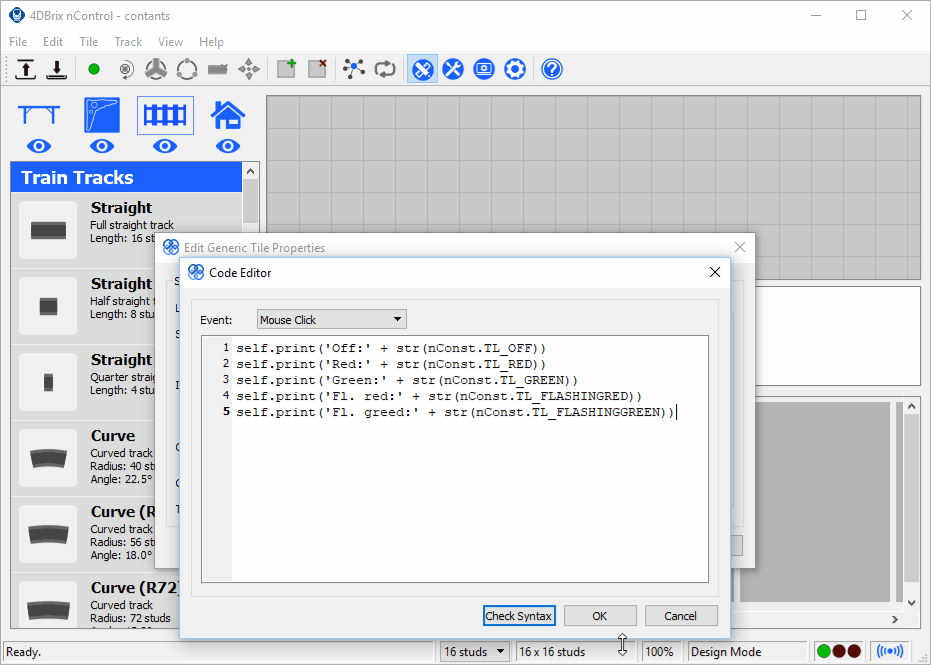Documentation > Python
 Constants What will you learn? You will learn what constants are. You will learn how you can use constants in your programs. What will your program do? It will show the constants associated with the states of a traffic light tile. It will show the values of the traffic light constants. What will you need? required: nControl 2018.0 or higher. Introduction In programming constants are values that cannot change. Constants are like variables, but their value is preset and cannot be changed. One of the main goal of constants is to make your programs more readable. The advantage of using constants Let's start by showing why constants are useful. Let's illustrate this with a traffic light tile. A traffic light tile has a number of different states: the light can off, it can red, ... Let's use a generic tile to print the values of the different state of the traffic light tile on our screen. The project below has two tiles: a traffic light tile and a generic tile. Start by downloading the project using the link below and opening it in nControl. The open the code editor of the generic tile:We'll start by creating a variable to access the traffic light tile. We can do this by calling the getTile() function of the generic tile. `light = self.getTile('Light 1')` Next, we going to request the current state of the traffic light and store that in the variable color. We can do this with the getState() function of the traffic light tile: `color = light.getState()` And finally, we're going to print the value of the variable color on our screen: `self.print(color)` So our full Python program becomes: `light = self.getTile('Light 1')color = light.getState()self.print(color)` When your program is complete, close code editor.To run the program, go to Simulation Mode, put the traffic light in the desired state and click the generic tile to show the value of the state. You can do this all the state of the traffic light:You can see that the various states are represented by numbers: off = 1, red = 2, green = 3, ... A computer program usually uses numbers to represent different states or options because that's more efficient from both a computational and memory usage point of view. However, for humans numbers are not very practical. It's hard to remember what 1, 2, 3, ... represents, strings (= text) would be a lot easier. And that's what constants are used for. If we, for example, define a constant with the name RED and give it the value 2, we can use RED instead of 2; and that a lot easier to read/remember. Printing the values of the constants nControl has a set of predefined constants for all the states of the traffic light. In nControl, all the constants are stored in an object called nConst. Their names also have a fixed structure fixed structure @@_@@@@@@@. The first two characters describe the type of the constant; for example, traffic light tile constants start with TL. The past after the underscore describes the state or option; for example, a red traffic light is represented by RED. So the full name of the constant describing the red state is: `nConst.TL_RED` The documentation of the traffic light tile shows that there are 5 constants: nConst.TL_FLASHINGGREEN nConst.TL_FLASHINGRED nConst.TL_GREEN nConst.TL_OFF nConst.TL_RED Now, let's print the values of these five traffic light constants. Open the code editor of the generic tile, remove the code and replace it with the a program:We can print the value of the nConst.TL_OFF with the following code: `self.print('Off:' + str(nConst.TL_OFF))` Let's look at this line in detail. To print text on our screen, we need to use the print() function of the tile that we're working in. That gives the code: `self.print()` In front of the value of the constant we would like to add some text to indicate which constant we're printing. In Python text has to be put between quotes. So that gives: `self.print('Off')` Now we need to add the value of the constant. The print() function has one argument, so we need to add the value of the constant to the string 'Off'. In Python you can combine strings (= text) with the + operator. So we get: `self.print('Off' + )` The nControl constants are integers (= whole numbers). You cannot combine a string and a number, so we need to convert the value of the constant to a text before we can combine it with 'Off'. In Python this is done with the str() function. That's a function that converts the provided value into a string. So that explains our line of code: `self.print('Off' + str(nConst.TL_OFF)` We can print the other constants in a similar way. So the following code is what we need to print the values of all the traffic light constants constants: `self.print('Off:' + str(nConst.TL_OFF))self.print('Red:' + str(nConst.TL_RED))self.print('Green:' + str(nConst.TL_GREEN))self.print('Fl. red:' + str(nConst.TL_FLASHINGRED))self.print('Fl. greed:' + str(nConst.TL_FLASHINGGREEN))` You can run the code by going to Simulation Mode and clicking the generic tile:You can see that the values of the constants correspond with the values that were returned by the getState() function. In the next lesson we will see how the use these constants in conditional expression so we can make our program do different things in function of the state of a tile. Constants in Python Python actually doesn't have the concept of constants, it only has variables. So the work around is the create variables, initialize them with the desired value and use them like they were constants. The main difference with real constants is that value of constants cannot be changed. As Python uses variables as constants you can actually change their value. So, in Python, you have to make sure that your code does alter the values of the 'constants'. So you should never use a line of code like: `nConst.TL_OFF = ...` Changing the value of constants will make your code behave in an unexpected way as it will start misinterpreting the values representing the states and options.
Documentation > Python > Lesson 5